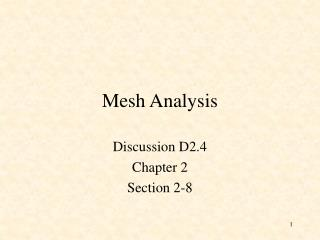DownloadDownload PresentationMesh Analysis

Mesh Analysis

Télécharger la présentationMesh Analysis

- - - - - - - - - - - - - - - - - - - - - - - - - - - E N D - - - - - - - - - - - - - - - - - - - - - - - - - - -
Presentation Transcript

1. Mesh Analysis Discussion D2.4 Chapter 2 Section 2-8

2. Mesh Analysis • Mesh analysis applies KVL to find unknown currents. • It is only applicable to planar circuits (a circuit that can be drawn on a plane with no branches crossing each other). • A mesh is a loop that does not contain any other loops. • The current through a mesh is known as the mesh current. • Assume for simplicity that the circuit contains only voltage sources.

3. Mesh Analysis Steps • Assign mesh currents i1, i2, i3, … il, to the l meshes, • Apply KVL to each of the l meshes and use Ohm’s law to express the voltages in terms of the mesh currents, • Solve the l resulting simultaneous equations to find the mesh currents.

4. Example 7 Number of nodes, n = 4 Number of loops, l = 10 Number of branches, b =

5. Example Apply KVL to each mesh Mesh 1: Mesh 2: Mesh 3: Mesh 4:

6. Mesh 1: Mesh 2: Mesh 3: Mesh 4: Express the voltage in terms of the mesh currents: Mesh 1: Mesh 2: Mesh 3: Mesh 4:

7. Mesh 1: Mesh 2: Mesh 3: Mesh 4: Mesh 1: Mesh 2: Mesh 3: Mesh 4:

8. Mesh 1: Mesh 2: Mesh 3: Mesh 4:

9. is an lxlsymmetric resistance matrix is a 1 xl vector of mesh currents is a vector of voltages representing “known” voltages

10. Writing the Mesh Equations by Inspection • The matrix R is symmetric, rkj = rjk and all of the off-diagonal terms are negative or zero. The rkk terms are the sum of all resistances in mesh k. The rkj terms are the negative sum of the resistances common to BOTH mesh k and mesh j. The vk (the kth component of the vector v) = the algebraic sum of the independent voltages in mesh k, with voltage rises taken as positive.

11. MATLAB Solution of Mesh Equations

12. Test with numbers

13. Test with numbers

14. MATLAB Run

15. PSpice Simulation MATLAB:

16. What happens if we have independent current sources in the circuit? • Assume the voltage across each current source is known. • Write the mesh equations in the same way we did for circuits with only independent or dependent voltage sources. • Express the current of each independent current source in terms of the mesh currents. • Rewrite the equations with all unknown mesh currents on the left hand side of the equality and all known voltages on the r.h.s. of the equality.

17. Example Write mesh equations by inspection.

18. MATLAB Run i1 A i2 A va V

19. PSpice Simulation va - + i2 i1 i1 MATLAB: i2 va

20. Nodal Analysis vs Mesh Analysis • If the circuit is nonplanar we must use nodal analysis. • If the circuit is planar, there are two principle considerations: • The number of equations we need to write. • The information we need in the circuit. For example, do we want to find a current or a voltage?

21. The Number of Mesh Equations If the circuit has only voltage sources or current sources in parallel with resistances (that is, current sources that can be exchanged for voltage sources) then after we have exchanged the current sources, we have to write l mesh equations and the fundamental theorem of network topology tells us that:

22. The Number of Nodal Equations We also know that if the circuit has only current sources or voltage sources in series with resistances (that is, sources that can be transformed to current sources) then after we have transformed the voltage sources, the number of nodal equations is (n - 1). If we are not interested in the currents through voltage sources or voltages across current sources we may be able to reduce the number of equations we have to write.

23. Example Transform voltage sources into current sources b = 7 n = 3

24. Example Write nodal equations

25. Example

26. Example

27. Example Transform current source into a current source and write mesh equations

28. Example

29. Example

30. Example